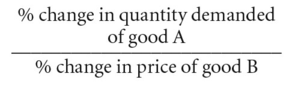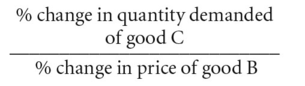cross-elasticity of demand

cross-elasticity of demand

see ELASTICITY OF DEMAND.

cross-elasticity of demand

a measure of the degree of responsiveness of the DEMAND for one good to a given change in the PRICE of some other good.Products may be regarded by consumers as substitutes for one another, in which case a rise in the price of good B (tea, for example) will tend to increase the quantity demanded of good A (coffee, for example). Here the cross-elasticity of demand will be positive since as the price of B goes up the quantity demanded of A rises as consumers now buy more A in preference to the more expensive B.Alternatively, products may be regarded by consumers as complements that are jointly demanded, in which case a rise in the price of good B (tea, for example) will tend to decrease not only the quantity demanded of good B but also another good, C (sugar, for example). Here the cross-elasticity of demand will be negative since a rise in the price of B serves to reduce the quantity demanded of C.

The degree of substitutability between products is reflected in the magnitude of the cross-elasticity measure. If a small increase in the price of good B results in a large rise in the quantity demanded of good A (highly cross-elastic), then goods B and A are close substitutes. Likewise, the degree of complementarity of products is reflected in the magnitude of the cross-elasticity measure. If a small increase in the price of good B results in a large fall in the quantity demanded of good C (highly cross-elastic), then goods C and B are close complements.

Cross-elasticities provide a useful indication of the substitutability of products, so helping to indicate the boundaries between markets. A group of products with high cross-elasticities of demand constitutes a distinct market, whether or not they share common technical characteristics; for example, mechanical and electronic watches are regarded by consumers as close substitutes. See MARKET.

References in periodicals archive ?
441, 453 (1964) ("Interchangeability of use and cross-elasticity of demand are not to be used to obscure competition but to 'recognize competition where, in fact, competition exists.
10-3458) ("'the outer boundaries of a product market are determined by the reasonable interchangeability of use or the cross-elasticity of demand between the product itself and substitutes for it'" (quoting Brown Shoe Co.
18, 2004) ("It is insufficient for an expert to merely mention cross-elasticity of demand or supply; an analysis is required.
The cross-elasticity of demand between primary care and pediatric services appears to be low enough to classify them into two distinct markets.
Again, one ideally would like to use a cross-elasticity of demand measure to observe the extent that patients will travel to visit physicians when there are relative changes in physician fees.
D] denote the market cross-elasticity of demand and [[epsilon].
The most important is that cross-elasticity of demand is constrained in size by the direct price elasticity.
Finally, cross-elasticity of demand or price concepts are useless since no quantitative standard is implied by economic theory.
In Brown Shoe, the Court held that the outer boundaries of a product market are determined by the reasonable interchangeability of use or cross-elasticity of demand between the product itself and substitutes for it,(8) and the "cross-elasticity of production facilities may also be an important factor in defining a product market.
35) The Court went on to elaborate that, "[i]f a slight decrease in the price of cellophane causes a considerable number of customers of other flexible wrappings to switch to cellophane, it would be an indication that a high cross-elasticity of demand exists between them; that the products compete in the same market.
Formally, cross-elasticity of demand is defined as the percentage change in quantity demanded for one good resulting from the percentage change in price of another good.
Assume also for this case that the cross-elasticity of demand between meat and poultry is one so that the three meat substitutes have a combined cross-elasticity of five.

Site: Follow: Share:
Open / Close